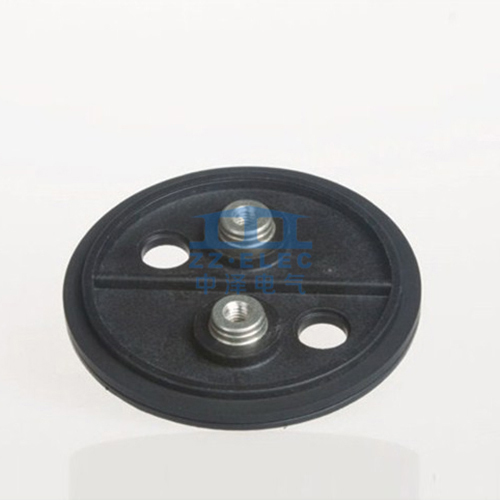# What are the main parameters of the capacitor?

Update:22,Mar,2021
Summary: (1) Nominal capacitance is the capacitance marked on the capacitor. But the act...
(1) Nominal capacitance is the capacitance marked on the capacitor. But the actual capacitance of the capacitor is
The nominal capacitance is deviated, and the accuracy level corresponds to the allowable error. Generally, capacitors are commonly used in grades Ⅰ, Ⅱ, and Ⅲ, and electrolytic capacitors use grades Ⅳ, Ⅴ, and Ⅵ to indicate capacity accuracy, which is selected according to the purpose. The capacitance value of an electrolytic capacitor depends on the impedance presented when working under AC voltage. The capacitance value will change with the change of operating frequency, temperature, voltage and measurement method. The unit of electric capacity is F (French).Since the capacitor is a kind of "container" for storing electric charge, there is a problem of the size of the "capacity". In order to measure the capacity of a capacitor to store charge, the physical quantity of capacitance is determined. Capacitors can store charge only under the action of applied voltage. The amount of charge stored by different capacitors under the action of voltage may also be different. Internationally, it is uniformly stipulated that when a 1 volt DC voltage is applied to a capacitor, the amount of charge it can store is the capacitance of the capacitor (that is, the amount of electricity per unit voltage), which is represented by the letter C. The basic unit of electric capacity is farad (F). Under the action of 1 volt DC voltage, if the charge stored in the capacitor is 1 coulomb, the capacitance is set as 1 farad, and the farad is represented by the symbol F, 1F=1Q/V. In practical applications, the capacitance of a capacitor is often much smaller than 1 farad, and smaller units are commonly used, such as millifarad (mF), microfarad (μF), nanofarad (nF), picofarad (pF), etc. The relationship is: 1 microfarad is equal to one millionth of a farad; 1 picofarad is equal to one millionth of a microfarad, that is:
1 farad (F)=1000 millifarads (mF); 1 millifarads (mF)=1000 microfarads (μF); 1 microfarad (μF)=1000 nanofarads (nF); 1 nanofarad (nF)=1000 skins Method (pF); namely: 1F=1000000μF; 1μF=1000000pF.
(2) The rated voltage is the highest DC voltage that can be continuously applied to the capacitor at the lowest ambient temperature and the rated ambient temperature. If the working voltage exceeds the withstand voltage of the capacitor, the capacitor will be broken down and cause damage. In practice, as the temperature increases, the withstand voltage value will become lower.
(3) Insulation resistance. The DC voltage is applied to the capacitor, and the leakage current is generated. The ratio of the two is called the insulation resistance. When the capacitance is small, its value mainly depends on the surface state of the capacitor; when the capacitance is greater than 0.1μF, its value mainly depends on the medium. Generally, the larger the insulation resistance, the better.
(4) Loss. Under the action of an electric field, the energy consumed by a capacitor in a unit of time due to heat is called loss. The loss is related to the frequency range, medium, conductance, and resistance of the metal part of the capacitor.
(5) Frequency characteristics. As the frequency increases, the capacitance of general capacitors shows a decreasing law. When the capacitor works below the resonant frequency, it is capacitive; when it exceeds its resonant frequency, it is inductive. At this time, it is not a capacitor but an inductance. Therefore, it is necessary to prevent the capacitor from operating above the resonant frequency.